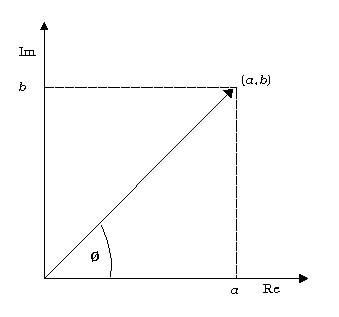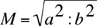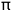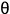# The FFT: Real, Imaginary, Magnitude, Phase

We’ve mentioned that most FFTs return their information in the form of a complex pair: a real part and an imaginary part. There’s obviously a lot more to say about that, but it gets pretty mathematical, and we’ll let you explore that on your own.

But we’ve been talking about the data that an FFT returns more in terms of the amplitude (or magnitude) and phase of a given frequency bin. What is important in this discussion is that you understand more or less how to get from the complex number pair to the magnitude/phase pair, which is generally, for computer music, more useful. You’ll probably want to manipulate amplitudes and phases, not complex numbers.

It turns out not to be so hard. Remember that an imaginary number, written in the form: a + bi, can be represented in a two-dimensional space as follows:Figure 1

The length of the line from the origin to the point (a, b) is the magnitude specific to the spectral bin; that is, it’s the amount of energy at that frequency. It’s just the hypotenuse of the right triangle drawn to the x-axis. The equation for it is very simple:You might recognize that as the equation for the length of the hypotenuse of a right triangle (called the Pythagorean theorem).

That was pretty easy, right? Deriving the phase from the complex pair is a little trickier. What we want to find is the angle made by that point to the x-axis. Remember that phases are expressed in radians, 0 to 2, and that a complete circle is 2. We call that angle. So, if we can findin radians, we’re done: we have the phase of that bin.

It turns out that we’re looking for an angle that is the ratio of the opposite side to the adjacent side (remember: SOH CAH TOA: sin, opposite over hypotenuse; cosine, adjacent over hypotenuse; tangent, opposite over adjacent). We want the angle whose tangent is the y-value (opposite) over the x-value (adjacent), or b/a. That’s called the arctangent, and is written as tan-1. So the equation for calculating phase is:= tan- 1 (b/a)

There, that wasn’t so painful, was it?